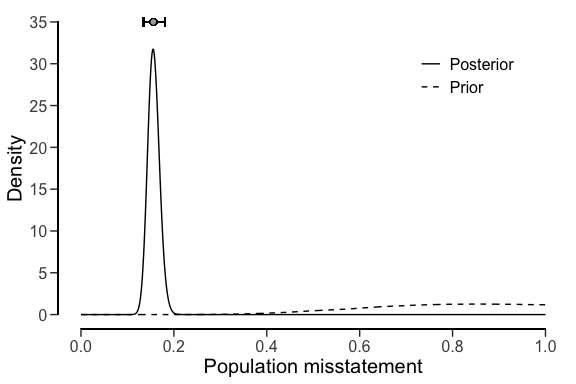# Evaluating statistical audit samples

## Introduction

This vignette demonstrates how to evaluate a sample with the evaluation() function from the jfa package.In auditing, the goal of the evaluation is typically to estimate the misstatement in the population based on a sample, or to test the misstatement in the population against a critical upper limit, referred to as the performance materiality.

## Required information

Evaluating an audit sample using the evaluation() function requires that the sample data is available in one of two forms:

• Summary statistics: This includes (a vector of) the number of items (n), (a vector of) the sum of errors / taints (x) and optionally (a vector of) the number of units in the population (N.units).
• Data: A data.frame containing a numeric column with book values (values), a numeric column with audit (true) values (values.audit) and optionally a factor column indicating stratum membership (strata).

By default, evaluation() estimates the population misstatement and returns a point estimate as well as a (conf.level $$\times$$ 100)% confidence / credible interval around this estimate. However, in audit sampling, the population is typically subject to a certain maximum tolerable misstatement defined by the performance materiality $$\theta_{max}$$. Using the materiality argument, you can provide this critical value to the evaluation() function as a fraction. In addition to the estimation that is performed by default, specifying materiality triggers the comparison of two competing hypotheses. Which hypotheses are being compared depends on the input for the alternative argument:

• alternative = "less" (default): $$H_1:\theta<\theta_{max}$$ versus $$H_0:\theta\geq\theta_{max}$$
• alternative = "greater": $$H_1:\theta>\theta_{max}$$ versus $$H_0:\theta\leq\theta_{max}$$
• alternative = "two.sided": $$H_1:\theta \neq\theta_{max}$$ versus $$H_0:\theta=\theta_{max}$$

## Evaluation using summary statistics

### Non-stratified samples

In a non-stratified sampling procedure, the auditor does not take samples from different strata or subgroups in a population. An example of such a situation would be where the auditor is auditing the general ledger of a small business.

#### Classical evaluation

Classical hypothesis testing uses the p-value to make a decision about whether to reject the hypothesis $$H_0$$ or not. As an example, consider that an auditor wants to verify whether the population contains less than 5 percent misstatement, implying the hypotheses $$H_1:\theta<0.05$$ and $$H_0:\theta\geq0.05$$. They have taken a sample of 100 items, of which 1 contained an error. They set the significance level for the p-value to 0.05, implying that $$p < 0.05$$ will be enough to reject the hypothesis $$H_0$$. The call below evaluates the sample using a classical non-stratified evaluation procedure.

evaluation(materiality = 0.05, x = 1, n = 100)
##
##  Classical Audit Sample Evaluation
##
## data:  1 and 100
## number of errors = 1, number of samples = 100, taint = 1, p-value =
## 0.040428
## alternative hypothesis: true misstatement rate is less than 0.05
## 95 percent confidence interval:
##  0.00000000 0.04743865
## most likely estimate:
##  0.01
## results obtained via method 'poisson'

The output shows that the most likely error in the population is estimated to be 1 / 100 = 1% and that the 95% (one-sided) confidence interval ranges from 0% to 4.74%. The output also shows that the p-value is lower than 0.05 implying that the hypothesis $$H_0$$ can be rejected. Hence, the auditor is able to conclude that the sample provides sufficient evidence to state with reasonable assurance that the population does not contain material misstatement.

#### Bayesian evaluation

Bayesian hypothesis testing uses the Bayes factor, $$BF_{10}$$ or $$BF_{01}$$, to make a statement about the evidence provided by the sample in support for one of the two hypotheses $$H_1$$ or $$H_0$$. As an example of how to interpret the Bayes factor, the value of $$BF_{10} = 10$$ (provided by the evaluation() function) can be interpreted as: the data are 10 times more likely to have occurred under the hypothesis $$H_1$$ than under the hypothesis $$H_0$$. $$BF_{10} > 1$$ indicates evidence in favor of $$H_1$$ and against $$H_0$$, while $$BF_{10} < 1$$ indicates evidence in favor of $$H_0$$ and against $$H_1$$. The evaluation() function returns the value for $$BF_{10}$$, but $$BF_{01}$$ can be computed as $$\frac{1}{BF_{10}}$$.

Consider the previous example of an auditor who wants to verify whether the population contains less than 5 percent misstatement, implying the hypotheses $$H_1:\theta<0.05$$ and $$H_0:\theta\geq0.05$$. They have taken a sample of 100 items, of which 1 was found to contain a misstatement. The prior distribution is assumed to be a default beta(1,1) prior. The call below evaluates the sample using a Bayesian non-stratified evaluation procedure.

prior <- auditPrior(materiality = 0.05, method = "default", likelihood = "binomial")
evaluation(materiality = 0.05, x = 1, n = 100, prior = prior)
##
##  Bayesian Audit Sample Evaluation
##
## data:  1 and 100
## number of errors = 1, number of samples = 100, taint = 1, BF₁₀ = 515.86
## alternative hypothesis: true misstatement rate is less than 0.05
## 95 percent credible interval:
##  0.00000000 0.04610735
## most likely estimate:
##  0.01
## results obtained via method 'binomial' + 'prior'

The output shows that the most likely error in the population is estimated to be 1 / 100 = 1% and that the 95% (one-sided) credible interval ranges from 0% to 4.61%. The small difference between the classical and default Bayesian results is due to the prior distribution, which must be proper in order to calculate a Bayes factor (classical results can be emulated by constructing a prior with method = "strict" in the auditPrior() function). The Bayes factor in this case is shown to be $$BF_{10}=515$$, meaning that the data from the sample are about 515 times more likely to occur under the hypothesis of tolerable misstatement than under the hypothesis of material misstatement.

Note that this is a very high Bayes factor for the little data that is observed. That is because the Bayes factor is dependent on the prior distribution for $$\theta$$. As a rule of thumb, when the prior distribution is highly conservative (as with method = 'default') with respect to the hypothesis of tolerable misstatement, the Bayes factor tends to over quantify the evidence in favor of this hypothesis. You can mitigate this dependency by using a prior distribution that is impartial with respect to the hypotheses via method = "impartial" in the auditPrior() function (Derks et al., 2022).

prior <- auditPrior(materiality = 0.05, method = "impartial", likelihood = "binomial")
evaluation(materiality = 0.05, x = 1, n = 100, prior = prior)
##
##  Bayesian Audit Sample Evaluation
##
## data:  1 and 100
## number of errors = 1, number of samples = 100, taint = 1, BF₁₀ = 47.435
## alternative hypothesis: true misstatement rate is less than 0.05
## 95 percent credible interval:
##  0.00000000 0.04110834
## most likely estimate:
##  0.0088878
## results obtained via method 'binomial' + 'prior'

The output shows that $$BF_{10}=47$$, implying that under the assumption of impartiality there is strong evidence for $$H_1$$, the hypothesis that the population contains misstatements lower than 5 percent of the population (tolerable misstatement). Since the two prior distributions both resulted in convincing Bayes factors, the results can be considered robust to the choice of prior distribution. Hence, the auditor is able to conclude that the sample provides convincing evidence to state that the population does not contain material misstatement.

### Stratified samples

In a stratified sampling procedure, the auditor takes samples from different strata (or subgroups) in a population. An example of such a situation would be a group audit where the audited organization consists of different components or branches. Stratification is relevant for the group auditor if they must form an opinion on the group as a whole because they must aggregate the samples taken by the component auditors.

As a data example, consider the retailer data set that comes with the package. The organization in question consists of 20 branches across the country. In each of the 20 strata, a component auditor has taken a statistical sample and reported the outcomes to the group auditor.

data(retailer)
print(retailer)
##    stratum items samples errors
## 1        1  5000     300     21
## 2        2  5000     300     16
## 3        3  5000     300     15
## 4        4  5000     300     14
## 5        5  5000     300     16
## 6        6  5000     150      5
## 7        7  5000     150      4
## 8        8  5000     150      3
## 9        9  5000     150      4
## 10      10  5000     150      5
## 11      11 10000      50      2
## 12      12 10000      50      3
## 13      13 10000      50      2
## 14      14 10000      50      1
## 15      15 10000      50      0
## 16      16 10000      15      0
## 17      17 10000      15      0
## 18      18 10000      15      0
## 19      19 10000      15      1
## 20      20  4000      15      3

In general, there are three approaches to evaluating a stratified sample: no pooling, complete pooling, and partial pooling (see Derks et al., 2022). When using evaluation(), you must to indicate which type of pooling to use via the pooling argument. No pooling assumes no similarities between strata, which means that all strata are analyzed independently. Complete pooling assumes no difference between strata, which means that all data is aggregated and analyzed as a whole. Finally, partial pooling assumes differences and similarities between strata, which means that information can be shared between strata. Partial pooling (i.e., multilevel/hierarchical modeling) is a powerful technique that can result in more efficient population and stratum estimates but is currently only feasible when performing a Bayesian analysis. For this reason, this vignette only describes the Bayesian approach to stratified evaluation but going from this approach to a classical approach only requires setting prior = FALSE.

The number of units per stratum in the population can be provided with N.units to weigh the stratum estimates to determine population estimate. This is called poststratification. If N.units is not specified, each stratum is assumed to be equally represented in the population.

#### Approach 1: No pooling

No pooling (pooling = "none", default) assumes no similarities between strata. This means that the prior distribution specified through prior is applied independently for each stratum. This allows for independent estimates for the misstatement in each stratum but also results in a relatively high uncertainty in the population estimate. The call below evaluates the sample using a Bayesian stratified evaluation procedure, in which the stratum estimates are poststratified to arrive at the population estimate.

set.seed(1) # Important because the posterior distribution is determined via sampling
result_np <- evaluation(
materiality = 0.05, method = "binomial", prior = TRUE,
n = retailer$samples, x = retailer$errors, N.units = retailer$items, alternative = "two.sided", pooling = "none" ) summary(result_np) ## ## Bayesian Audit Sample Evaluation Summary ## ## Options: ## Confidence level: 0.95 ## Population size: 144000 ## Materiality: 0.05 ## Hypotheses: H₀: Θ = 0.05 vs. H₁: Θ ≠ 0.05 ## Method: binomial ## Prior distribution: Nonparametric ## ## Data: ## Sample size: 2575 ## Number of errors: 115 ## Sum of taints: 115 ## ## Results: ## Posterior distribution: Nonparametric ## Most likely error: 0.0598 ## 95 percent credible interval: [0.042763, 0.082201] ## Precision: 0.022401 ## BF₁₀: 0 ## ## Strata (20): ## N n x t mle lb ub precision ## 1 5000 300 21 21 0.07000 0.04637 0.10467 0.03467 ## 2 5000 300 16 16 0.05333 0.03324 0.08489 0.03156 ## 3 5000 300 15 15 0.05000 0.03069 0.08086 0.03086 ## 4 5000 300 14 14 0.04667 0.02816 0.07681 0.03014 ## 5 5000 300 16 16 0.05333 0.03324 0.08489 0.03156 ## 6 5000 150 5 5 0.03333 0.01472 0.07558 0.04225 ## 7 5000 150 4 4 0.02667 0.01084 0.06643 0.03977 ## 8 5000 150 3 3 0.02000 0.00726 0.05696 0.03696 ## 9 5000 150 4 4 0.02667 0.01084 0.06643 0.03977 ## 10 5000 150 5 5 0.03333 0.01472 0.07558 0.04225 ## 11 10000 50 2 2 0.04000 0.01230 0.13459 0.09459 ## 12 10000 50 3 3 0.06000 0.02178 0.16242 0.10242 ## 13 10000 50 2 2 0.04000 0.01230 0.13459 0.09459 ## 14 10000 50 1 1 0.02000 0.00478 0.10447 0.08447 ## 15 10000 50 0 0 0.00000 0.00050 0.06978 0.06978 ## 16 10000 15 0 0 0.00000 0.00158 0.20591 0.20591 ## 17 10000 15 0 0 0.00000 0.00158 0.20591 0.20591 ## 18 10000 15 0 0 0.00000 0.00158 0.20591 0.20591 ## 19 10000 15 1 1 0.06667 0.01551 0.30232 0.23565 ## 20 4000 15 3 3 0.20000 0.07266 0.45646 0.25646 In this case, the output of the summary() function shows that the estimate of the misstatement in the population is 5.85%, with the 95% credible interval ranging from 4.28% to 8.22%. The stratum estimates differ substantially from each other but are relatively uncertain. plot(result_np, type = "estimates")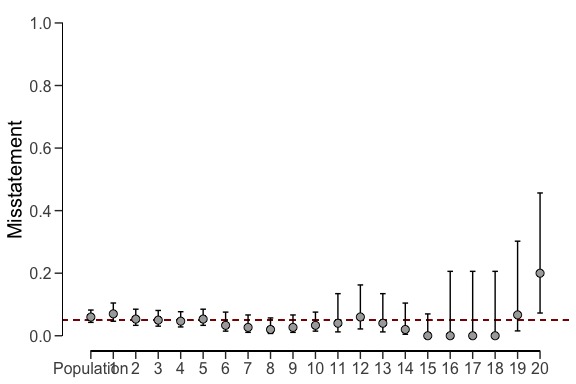The prior and posterior distribution for the population misstatement can be requested via the plot() function. plot(result_np, type = "posterior")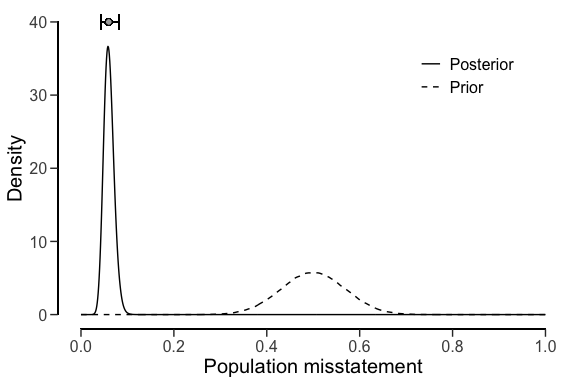#### Approach 2: Complete pooling Complete pooling (pooling = "complete") assumes no differences between strata. This has the advantages that data from all strata can be aggregated, which decreases the uncertainty in the population estimate compared to the no pooling approach. However, the disadvantage of this approach is that it does not facilitate the distinction between between strata, as every stratum receives the same estimate equal to that of the population. The call below evaluates the sample using a Bayesian stratified evaluation procedure, in which the strata are assumed to be the same. result_cp <- evaluation( materiality = 0.05, method = "binomial", prior = TRUE, n = retailer$samples, x = retailer$errors, N.units = retailer$items,
alternative = "two.sided", pooling = "complete"
)
summary(result_cp)
##
##  Bayesian Audit Sample Evaluation Summary
##
## Options:
##   Confidence level:               0.95
##   Population size:                144000
##   Materiality:                    0.05
##   Hypotheses:                     H₀: Θ = 0.05 vs. H₁: Θ ≠ 0.05
##   Method:                         binomial
##   Prior distribution:             beta(α = 1, β = 1)
##
## Data:
##   Sample size:                    2575
##   Number of errors:               115
##   Sum of taints:                  115
##
## Results:
##   Posterior distribution:         beta(α = 116, β = 2461)
##   Most likely error:              0.04466
##   95 percent credible interval:   [0.03735, 0.053345]
##   Precision:                      0.0086852
##   BF₁₀:                            0.022725
##
## Strata (20):
##        N   n  x  t     mle      lb      ub precision    bf10
## 1   5000 300 21 21 0.04466 0.03735 0.05335   0.00869 0.02273
## 2   5000 300 16 16 0.04466 0.03735 0.05335   0.00869 0.02273
## 3   5000 300 15 15 0.04466 0.03735 0.05335   0.00869 0.02273
## 4   5000 300 14 14 0.04466 0.03735 0.05335   0.00869 0.02273
## 5   5000 300 16 16 0.04466 0.03735 0.05335   0.00869 0.02273
## 6   5000 150  5  5 0.04466 0.03735 0.05335   0.00869 0.02273
## 7   5000 150  4  4 0.04466 0.03735 0.05335   0.00869 0.02273
## 8   5000 150  3  3 0.04466 0.03735 0.05335   0.00869 0.02273
## 9   5000 150  4  4 0.04466 0.03735 0.05335   0.00869 0.02273
## 10  5000 150  5  5 0.04466 0.03735 0.05335   0.00869 0.02273
## 11 10000  50  2  2 0.04466 0.03735 0.05335   0.00869 0.02273
## 12 10000  50  3  3 0.04466 0.03735 0.05335   0.00869 0.02273
## 13 10000  50  2  2 0.04466 0.03735 0.05335   0.00869 0.02273
## 14 10000  50  1  1 0.04466 0.03735 0.05335   0.00869 0.02273
## 15 10000  50  0  0 0.04466 0.03735 0.05335   0.00869 0.02273
## 16 10000  15  0  0 0.04466 0.03735 0.05335   0.00869 0.02273
## 17 10000  15  0  0 0.04466 0.03735 0.05335   0.00869 0.02273
## 18 10000  15  0  0 0.04466 0.03735 0.05335   0.00869 0.02273
## 19 10000  15  1  1 0.04466 0.03735 0.05335   0.00869 0.02273
## 20  4000  15  3  3 0.04466 0.03735 0.05335   0.00869 0.02273

For example, the output of the summary() function shows that the estimate of the misstatement in the population is 4.47%, with the 95% credible interval ranging from 3.74% to 5.33%. Since the data is aggregated, the stratum estimates contain relatively little uncertainty. However, the probability of misstatement in stratum 20 (many misstatements) under this assumption is the same as that of stratum 15 (few misstatements).

plot(result_cp, type = "estimates")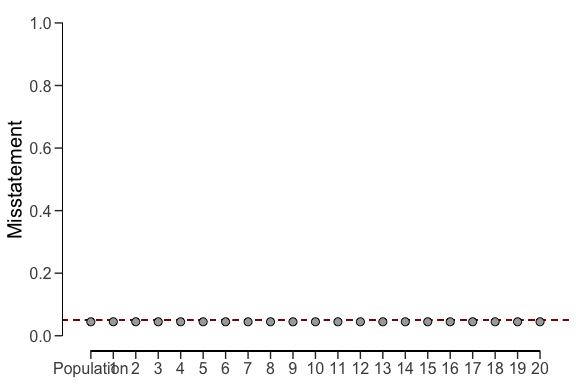The prior and posterior distribution for the population misstatement can be requested via the plot() function.

plot(result_cp, type = "posterior")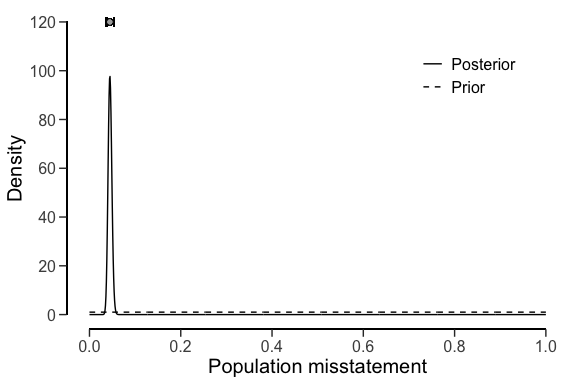#### Approach 3: Partial pooling

Finally, partial pooling (pooling = "partial") assumes differences and similarities between strata. This allows the auditor to differentiate between strata, while also sharing information between the strata to reduce uncertainty in the population estimate. The call below evaluates the sample using a Bayesian stratified evaluation procedure, in which the stratum estimates are poststratified to arrive at the population estimate.

set.seed(1) # Important because the posterior distribution is determined via sampling
result_pp <- evaluation(
materiality = 0.05, method = "binomial", prior = TRUE,

#### Classical evaluation

The call below evaluates the allowances sample using a classical stratified evaluation procedure, in which the stratum estimates are poststratified to arrive at the population estimate.

x <- evaluation(
materiality = 0.05, data = allowances,
values = "bookValue", values.audit = "auditValue", strata = "branch", times = "times",
alternative = "two.sided", N.units = N.units
)
summary(x)
##
##  Classical Audit Sample Evaluation Summary
##
## Options:
##   Confidence level:               0.95
##   Population size:                16772249
##   Materiality:                    0.05
##   Hypotheses:                     H₀: Θ = 0.05 vs. H₁: Θ ≠ 0.05
##   Method:                         poisson
##
## Data:
##   Sample size:                    1604
##   Number of errors:               401
##   Sum of taints:                  252.9281046
##
## Results:
##   Most likely error:              0.14723
##   95 percent confidence interval: [0.12549, 0.18239]
##   Precision:                      0.03516
##   p-value:                        NA
##
## Strata (16):
##             N   n   x         t     mle      lb      ub precision p.value
## 1   317200.09  87   6   1.27814 0.01469 0.00073 0.06950   0.05481 0.46285
## 2  2792814.33 305 233 193.23313 0.63355 0.54558 0.72945   0.09590 0.00000
## 3  1144231.69  55   3   3.00000 0.05455 0.01105 0.15940   0.10486 0.75827
## 4   414202.89  70  45  15.05094 0.21501 0.11878 0.35434   0.13933 0.00000
## 5    96660.53  18   1   0.64537 0.03585 0.00015 0.27456   0.23871 0.59343
## 6   348006.13  34   1   0.17866 0.00525 0.00000 0.11926   0.11401 1.00000
## 7  2384079.33  55  14   9.44448 0.17172 0.07885 0.32122   0.14950 0.00058
## 8  1840399.33  96   1   0.00813 0.00008 0.00000 0.03860   0.03852 0.10355
## 9   563957.70  92   0   0.00000 0.00000 0.00000 0.04010   0.04010 0.01783
## 10 3198877.73 201   7   0.92023 0.00458 0.00009 0.02703   0.02245 0.00122
## 11 1983299.06 128   7   1.50034 0.01172 0.00084 0.05013   0.03841 0.10773
## 12  319144.13  86   5   1.68141 0.01955 0.00174 0.07806   0.05851 0.46069
## 13  148905.79  25   0   0.00000 0.00000 0.00000 0.14756   0.14756 0.64187
## 14  513058.76 150   0   0.00000 0.00000 0.00000 0.02459   0.02459 0.00134
## 15  432007.61 150  39  21.80000 0.14533 0.09026 0.22045   0.07511 0.00001
## 16  275403.70  52  39   4.18726 0.08052 0.02237 0.20215   0.12163 0.12258

In this case, the output shows that the estimate of the misstatement in the population is 14.72%, with the 95% confidence interval ranging from 12.55% to 18.26%. The precision of the population estimate is 3.54%. The stratum estimates can be seen in the output of the summary() function and are visualized below.

plot(x, type = "estimates")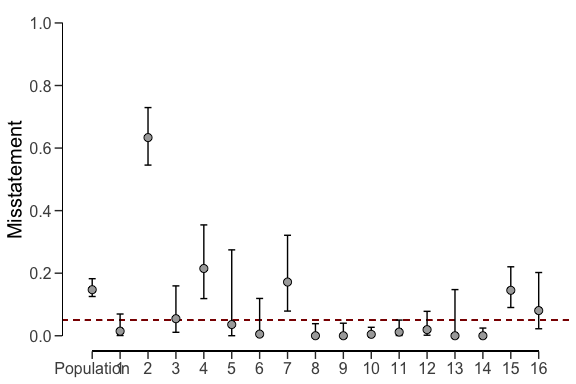#### Bayesian evaluation

Bayesian inference can improve upon the estimates of the classical approach by pooling information between strata where possible. The call below evaluates the allowances sample using a Bayesian stratified evaluation procedure, in which the stratum estimates are poststratified to arrive at the population estimate.

x <- evaluation(
materiality = 0.05, data = allowances, prior = TRUE,
values = "bookValue", values.audit = "auditValue", strata = "branch", times = "times",
alternative = "two.sided", N.units = N.units
)
summary(x)
##
##  Bayesian Audit Sample Evaluation Summary
##
## Options:
##   Confidence level:               0.95
##   Population size:                16772249
##   Materiality:                    0.05
##   Hypotheses:                     H₀: Θ = 0.05 vs. H₁: Θ ≠ 0.05
##   Method:                         poisson
##   Prior distribution:             Nonparametric
##
## Data:
##   Sample size:                    1604
##   Number of errors:               401
##   Sum of taints:                  252.9281046
##
## Results:
##   Posterior distribution:         Nonparametric
##   Most likely error:              0.1558
##   95 percent credible interval:   [0.13473, 0.1813]
##   Precision:                      0.025505
##   BF₁₀:                            NaN
##
## Strata (16):
##             N   n   x         t     mle      lb      ub precision
## 1   317200.09  87   6   1.27814 0.01452 0.00380 0.06871   0.05419
## 2  2792814.33 305 233 193.23313 0.63148 0.54862 0.72707   0.09559
## 3  1144231.69  55   3   3.00000 0.05357 0.01946 0.15656   0.10299
## 4   414202.89  70  45  15.05094 0.21199 0.12935 0.34935   0.13736
## 5    96660.53  18   1   0.64537 0.03397 0.00749 0.26011   0.22614
## 6   348006.13  34   1   0.17866 0.00510 0.00137 0.11585   0.11075
## 7  2384079.33  55  14   9.44448 0.16865 0.09112 0.31548   0.14683
## 8  1840399.33  96   1   0.00813 0.00008 0.00027 0.03821   0.03812
## 9   563957.70  92   0   0.00000 0.00000 0.00027 0.03967   0.03967
## 10 3198877.73 201   7   0.92023 0.00456 0.00108 0.02689   0.02234
## 11 1983299.06 128   7   1.50034 0.01163 0.00322 0.04974   0.03811
## 12  319144.13  86   5   1.68141 0.01933 0.00559 0.07716   0.05784
## 13  148905.79  25   0   0.00000 0.00000 0.00097 0.14188   0.14188
## 14  513058.76 150   0   0.00000 0.00000 0.00017 0.02443   0.02443
## 15  432007.61 150  39  21.80000 0.14437 0.09550 0.21899   0.07462
## 16  275403.70  52  39   4.18726 0.07900 0.03262 0.19834   0.11933

The output shows that the estimate of the misstatement in the population is 15.66%, with the 95% credible interval ranging from 14.59% to 17%. The precision of the population estimate is 1.34%, which is substantially lower than that of the classical approach. The stratum estimates can be seen in the output of the summary() function and are visualized below.

plot(x, type = "estimates")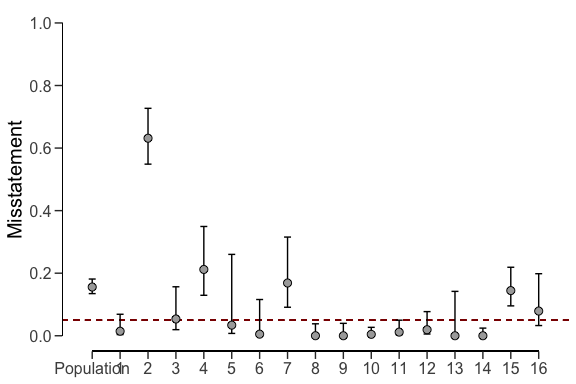The prior and posterior distribution for the population misstatement can be requested via the plot() function.

plot(x, type = "posterior")